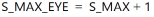# Logic Optimization

## Clock and Data Recovery Unit based on Deserialized Oversampled Data (XAPP1240)

Document ID
XAPP1240
Release Date
2022-11-04
Revision
3.1 English

The NIDRU performs many calculations during runtime. The precision of these calculations is controlled by the MASK_CG attribute, which permits the ability to trade off between precision and resource usage. For example:

MASK_CG = 1111111111111111 sets calculations to internal 16-bit precision (highest precision, highest resource usage)

MASK_CG = 1111111111111110 sets calculations to internal 15-bit precision (lower precision, lower resource usage)

TIP:   Lowering the internal precision to 10-bit has a negligible impact on the performance.

Reducing the internal calculation precision negatively impacts the jitter tolerance. The amount has to be evaluated either in hardware or in simulation.

S_MAX is the expected maximum number of extracted samples per clock cycle. NMAX is the maximum number of bits processed per clock cycle.

IMPORTANT:   It is mandatory to set S_MAX > N_MAX. N_MAX is calculated using the following equation:
N_MAX = truncate (fDIN/fREFCLK+1)

S_MAX_EYE is an internal parameter and must be set according to the following equation:

Equation 6